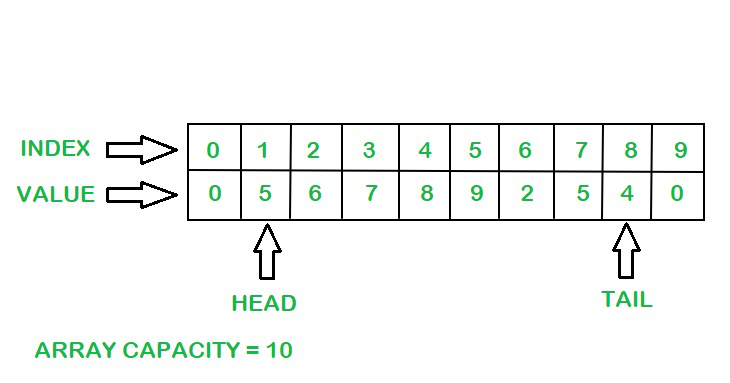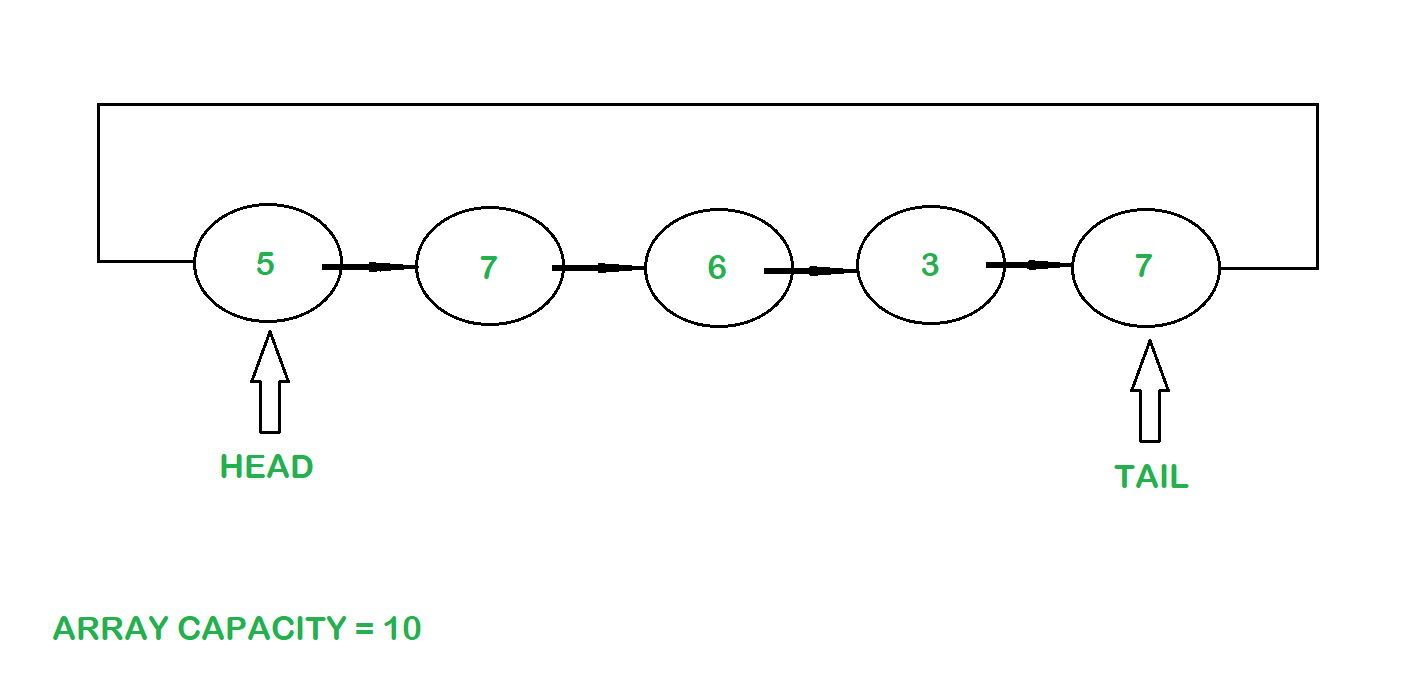Related Articles

# Java Program to Implement Circular Buffer

• Last Updated : 31 Aug, 2021

When data is constantly moved from one place to another or from one process to another or is frequently accessed, it cannot be stored in permanent memory locations such as hard drives as they take time to retrieve the data. This type of data needs to be accessed quickly and is stored in temporary memory locations such as RAM known as buffers.

Examples of Buffer:

• When any video is streamed online, the data (the audio and video)is buffered right before the video is played. During this buffering process, the data is downloaded and stored in the RAM and is accessed whenever needed.
• A Word document stores the content and the changes made by the user in a buffer before the document is saved.

What is a Circular Buffer?

Circular Buffer or Ring Buffer is a circular queue that allows the usage of memory in a contiguous manner. Circular Buffer follows the FIFO principle i.e First In First Out.

Circular Buffers can be implemented in two ways, using an array or a linked list.

Approach 1: Using an Array

An empty object array along with its capacity is initialized inside the constructor as the type of elements added is unknown. Two pointers namely head and tail are maintained for insertion and deletion of elements. The head points to the first element and the tail points to the last element.Circular Buffer using an array

Insertion of elements

Initially, the head is 0, the tail is -1 and the size is 0.

The index at which the element needs to be inserted is calculated using the formula: –

```int index = (tail + 1) % capacity
array[index] = element;```

The tail pointer and the size increment by one upon insertion of an element. When the size of the array becomes equal to its capacity, the buffer is full and no more elements can be accommodated.

Deletion of elements:

The element at the head pointer is retrieved and the head pointer is incremented by one and the size of the buffer if decremented by one.

```int index = head % capacity;
E element = (E) array[index];```

Example:

```Input : [5, 6, 7, 1 ,4]

Output : The elements are printed in the order :-
5
6
7
1
4```

Below is the implementation of the above approach

## Java

 `// Java program to implement a``// Circular Buffer using an array` `import` `java.io.*;``import` `java.lang.*;``class` `CircularBuffer {` `    ``// Initial Capacity of Buffer``    ``private` `int` `capacity = ``0``;``    ``// Initial Size of Buffer``    ``private` `int` `size = ``0``;``    ``// Head pointer``    ``private` `int` `head = ``0``;``    ``// Tail pointer``    ``private` `int` `tail = -``1``;``    ``private` `Object[] array;` `    ``// Constructor``    ``CircularBuffer(``int` `capacity)``    ``{``        ``// Initializing the capacity of the array``        ``this``.capacity = capacity;` `        ``// Initializing the array``        ``array = ``new` `Object[capacity];``    ``}` `    ``// Addition of elements``    ``public` `void` `add(Object element) ``throws` `Exception``    ``{` `        ``// Calculating the index to add the element``        ``int` `index = (tail + ``1``) % capacity;` `        ``// Size of the array increases as elements are added``        ``size++;` `        ``// Checking if the array is full``        ``if` `(size == capacity) {``            ``throw` `new` `Exception(``"Buffer Overflow"``);``        ``}` `        ``// Storing the element in the array``        ``array[index] = element;` `        ``// Incrementing the tail pointer to point``        ``// to the element added currently``        ``tail++;``    ``}` `    ``// Deletion of elements``    ``public` `Object get() ``throws` `Exception``    ``{` `        ``// Checking if the array is empty``        ``if` `(size == ``0``) {``            ``throw` `new` `Exception(``"Empty Buffer"``);``        ``}` `        ``// Calculating the index of the element to be``        ``// deleted``        ``int` `index = head % capacity;` `        ``// Getting the element``        ``Object element = array[index];` `        ``// Incrementing the head pointer to point``        ``// to the next element``        ``head++;` `        ``// Decrementing the size of the array as the``        ``// elements are deleted``        ``size--;` `        ``// Returning the first element``        ``return` `element;``    ``}` `    ``// Retrieving the first element without deleting it``    ``public` `Object peek() ``throws` `Exception``    ``{` `        ``// Checking if the array is empty``        ``if` `(size == ``0``) {``            ``throw` `new` `Exception(``"Empty Buffer"``);``        ``}` `        ``// Calculating the index of the``        ``// element to be deleted``        ``int` `index = head % capacity;` `        ``// Getting the element``        ``Object element = array[index];` `        ``// Returning the element``        ``return` `element;``    ``}` `    ``// Checking if the array is empty``    ``public` `boolean` `isEmpty() { ``return` `size == ``0``; }` `    ``// Size of the array``    ``public` `int` `size() { ``return` `size; }``}` `class` `Main {``    ``public` `static` `void` `main(String[] args) ``throws` `Exception``    ``{` `        ``// Creating the Circular Buffer``        ``CircularBuffer cb = ``new` `CircularBuffer(``10``);` `        ``// Adding elements to the circular Buffer``        ``cb.add(``5``);``        ``cb.add(``6``);``        ``cb.add(``7``);``        ``cb.add(``1``);``        ``cb.add(``4``);` `        ``// Printing the elements``        ``System.out.println(``            ``"The elements are printed in the order :-"``);``        ``System.out.println(cb.get());``        ``System.out.println(cb.get());``        ``System.out.println(cb.get());``        ``System.out.println(cb.get());``        ``System.out.println(cb.get());``    ``}``}`
Output
```The elements are printed in the order :-
5
6
7
1
4```

Time complexity: O(1), for both insertion and deletion.

Approach 2: Using a Linked List

A Generic Node class is created which acts as a helper class to create the Circular Buffer.

Two pointers namely head and tail are maintained for insertion and deletion of elements. The head points to the first element and the tail points to the last element.Insertion of elements:

• Initially the head and tail are null and the size is 0.
• Elements are added to the tail of the Linked List and the reference of the tail is changed to the head pointer.
• Size of the Buffer increases as elements are added into the Linked List.
• When the size of the array becomes equal to its capacity, the buffer is full and no more elements can be accommodated.

Deletion of elements:

The element at the head pointer is retrieved and the reference of the head pointer changes to the next element and the size of the buffer if decremented by one.

Example :

```Input : [5, 6, 7, 1 ,4]
Output: The elements are printed in the order :
5
6
7
1
4```

Below is the implementation of the above approach:

## Java

 `// Java program to implement a Circular``// Buffer using a Linked List` `// A Generic Node class is used to create a Linked List``class` `Node {``    ``// Data Stored in each Node of the Linked List``    ``E data;``    ``// Pointer to the next node in the Linked List``    ``Node next;` `    ``// Node class constructor used to initializes``    ``// the data in each Node``    ``Node(E data) { ``this``.data = data; }``}` `class` `CircularBufferLL {` `    ``// Head node``    ``Node head;` `    ``// Tail Node``    ``Node tail;``    ``int` `size = ``0``;``    ``int` `capacity = ``0``;` `    ``// Constructor``    ``CircularBufferLL(``int` `capacity)``    ``{``        ``this``.capacity = capacity;``    ``}` `    ``// Addition of Elements``    ``public` `void` `add(E element) ``throws` `Exception``    ``{` `        ``// Size of buffer increases as elements``        ``// are added to the Linked List``        ``size++;` `        ``// Checking if the buffer is full``        ``if` `(size == capacity) {``            ``throw` `new` `Exception(``"Buffer Overflow"``);``        ``}` `        ``// Checking if the buffer is empty``        ``if` `(head == ``null``) {``            ``head = ``new` `Node<>(element);``            ``tail = head;``            ``return``;``        ``}` `        ``// Node element to be linked``        ``Node temp = ``new` `Node<>(element);` `        ``// Referencing the last element to the head node``        ``temp.next = head;` `        ``// Updating the tail reference to the``        ``// latest node added``        ``tail.next = temp;` `        ``// Updating the tail to the latest node added``        ``tail = temp;``    ``}` `    ``// Retrieving the head element``    ``public` `E get() ``throws` `Exception``    ``{` `        ``// Checking if the buffer is empty``        ``if` `(size == ``0``) {``            ``throw` `new` `Exception(``"Empty Buffer"``);``        ``}``        ``// Getting the element``        ``E element = head.data;` `        ``// Updating the head pointer``        ``head = head.next;` `        ``// Updating the tail reference to``        ``// the new head pointer``        ``tail.next = head;` `        ``// Decrementing the size``        ``size--;``        ``if` `(size == ``0``) {``            ``// Removing any references present``            ``// when the buffer becomes empty``            ``head = tail = ``null``;``        ``}``        ``return` `element;``    ``}` `    ``// Retrieving the head element without deleting``    ``public` `E peek() ``throws` `Exception``    ``{` `        ``// Checking if the buffer is empty``        ``if` `(size == ``0``) {``            ``throw` `new` `Exception(``"Empty Buffer"``);``        ``}``        ``// Getting the element``        ``E element = head.data;``        ``return` `element;``    ``}` `    ``// Checking if the buffer is empty``    ``public` `boolean` `isEmpty() { ``return` `size == ``0``; }` `    ``// Retrieving the size of the buffer``    ``public` `int` `size() { ``return` `size; }``}` `class` `GFG {``    ``public` `static` `void` `main(String[] args) ``throws` `Exception``    ``{` `        ``// Creating the Circular Buffer``        ``CircularBufferLL cb``            ``= ``new` `CircularBufferLL<>(``10``);` `        ``// Adding elements to the circular Buffer``        ``cb.add(``5``);``        ``cb.add(``6``);``        ``cb.add(``7``);``        ``cb.add(``1``);``        ``cb.add(``4``);` `        ``// Printing the elements``        ``System.out.println(``            ``"The elements are printed in the order :-"``);``        ``System.out.println(cb.get());``        ``System.out.println(cb.get());``        ``System.out.println(cb.get());``        ``System.out.println(cb.get());``        ``System.out.println(cb.get());``    ``}``}`
Output
```The elements are printed in the order :-
5
6
7
1
4```

Time complexity: O(1), for both insertion and deletion.

Attention reader! Don’t stop learning now. Get hold of all the important Java Foundation and Collections concepts with the Fundamentals of Java and Java Collections Course at a student-friendly price and become industry ready. To complete your preparation from learning a language to DS Algo and many more,  please refer Complete Interview Preparation Course.

My Personal Notes arrow_drop_up